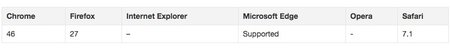## 【译】ES6中Arguments和Parameters用法解析

• July 20th, 2016

ECMAScript 6 (也称 ECMAScript 2015) 是ECMAScript 标准的最新版本，显著地完善了JS中参数的处理方式。除了其它新特性外，我们还可以使用rest参数、默认值、解构赋值等。

### 对比 Arguments 和 Parameters Link

``````function foo(param1, param2) {
// do something
}
foo(10, 20);
``````

``````var myArray = [5, 10, 50];
Math.max(myArray);    // Error: NaN
Math.max.apply(Math, myArray);    // 50
``````

`Math.max()` 方法并不支持传入数组，它只接受数字。所以当我们把数组作为参数传递给它时，就会抛出错误。但是，加上 `apply()` 方法后，数组会被转换成单独的数字，就能被 `Math.max()` 处理了。

``````var myArray = [5, 10, 50];
Math.max(...myArray);    // 50
``````

``````function myFunction() {
for(var i in arguments){
console.log(arguments[i]);
}
}
var params = [10, 15];
myFunction(5, ...params, 20, ...);    // 5 10 15 20 25
``````

``````new Date(...[2016, 5, 6]);    // Mon Jun 06 2016 00:00:00 GMT-0700 (Pacific Daylight Time)`
``````

``````new Date.apply(null, [2016, 4, 24]);    // TypeError: Date.apply is not a constructor
new (Function.prototype.bind.apply(Date, [null].concat([2016, 5, 6])));   // Mon Jun 06 2016 00:00:00 GMT-0700 (Pacific Daylight Time)
``````

rest参数和扩展操作符拥有相同的语法，不同的是，`rest参数是把所有的参数收集起来转换成数组`，而扩展操作符是把数组扩展成单独的参数。

``````function myFunction(...options) {
return options;
}
myFunction('a', 'b', 'c');      // ["a", "b", "c"]
``````

``````function myFunction(...options) {
return options;
}
myFunction();      // []
``````

rest参数在创建一个可变函数（即一个参数个数可变的函数）时尤其有用。rest参数有着数组固有的优势，可以快捷地替换 `arguments` 对象（下文会解释这个名词）。下面这个函数是用ES5写的，我们来看下：

``````function checkSubstrings(string) {
for (var i = 1; i < arguments.length; i++) {
if (string.indexOf(arguments[i]) === -1) {
return false;
}
}
return true;
}
checkSubstrings('this is a string', 'is', 'this');   // true
``````

``````function checkSubstrings(string, ...keys) {
for (var key of keys) {
if (string.indexOf(key) === -1) {
return false;
}
}
return true;
}
checkSubstrings('this is a string', 'is', 'this');   // true
``````

``````function logArguments(a, ...params, b) {
console.log(a, params, b);
}
logArguments(5, 10, 15);    // SyntaxError: parameter after rest parameter
``````

``````function logArguments(...param1, ...param2) {
}
logArguments(5, 10, 15);    // SyntaxError: parameter after rest parameter
``````

``````function foo(param1, param2) {
param1 = param1 || 10;
param2 = param2 || 10;
console.log(param1, param2);
}
foo(5, 5);  // 5 5
foo(5);    // 5 10
foo();    // 10 10
``````

``````function foo(param1, param2) {
if(param1 === undefined){
param1 = 10;
}
if(param2 === undefined){
param2 = 10;
}
console.log(param1, param2);
}
foo(0, null);    // 0, null
foo();    // 10, 10
``````

``````function foo(a = 10, b = 10) {
console.log(a, b);
}
foo(5);    // 5 10
foo(0, null);    // 0 null
``````

``````function getParam() {
return 3;
}
function multiply(param1, param2 = getParam()) {
return param1 * param2;
}
multiply(2, 5);     // 10
multiply(2);     // 6 (also displays an alert dialog)
``````

``````function myFunction(a=10, b=a) {
console.log('a = ' + a + '; b = '  + b);
}
myFunction();     // a=10; b=10
myFunction(22);    // a=22; b=22
myFunction(2, 4);    // a=2; b=4
``````

``````function myFunction(a, b = ++a, c = a*b) {
console.log(c);
}
myFunction(5);    // 36
``````

``````function add(value, array = []) {
array.push(value);
return array;
}
add(6);    // , not [5, 6]
``````

``````function initiateTransfer(options) {
var  protocol = options.protocol,
port = options.port,
delay = options.delay,
retries = options.retries,
timeout = options.timeout,
log = options.log;
// code to initiate transfer
}
options = {
protocol: 'http',
port: 800,
delay: 150,
retries: 10,
timeout: 500,
log: true
};
initiateTransfer(options);
``````

``````function initiateTransfer({protocol, port, delay, retries, timeout, log}) {
// code to initiate transfer
};
var options = {
protocol: 'http',
port: 800,
delay: 150,
retries: 10,
timeout: 500,
log: true
}
initiateTransfer(options);
``````

``````function initiateTransfer(param1, {protocol, port, delay, retries, timeout, log}) {
// code to initiate transfer
}
initiateTransfer('some value', options);
``````

``````function initiateTransfer({protocol, port, delay, retries, timeout, log}) {
// code to initiate transfer
}
initiateTransfer();  // TypeError: Cannot match against 'undefined' or 'null'
``````

``````function initiateTransfer({protocol, port, delay, retries, timeout, log} = {}) {
// code to initiate transfer
}
initiateTransfer();    // no error
``````

``````function initiateTransfer({
protocol = 'http',
port = 800,
delay = 150,
retries = 10,
timeout = 500,
log = true
}) {
// code to initiate transfer
}
``````

``````var a = 5;
function increment(a) {
a = ++a;
console.log(a);
}
increment(a);   // 6
console.log(a);    // 5
``````

``````function foo(param){
param.bar = 'new value';
}
obj = {
bar : 'value'
}
console.log(obj.bar);   // value
foo(obj);
console.log(obj.bar);   // new value
``````

• 实参少于形参

• 实参多于形参

``````function foo(mandatory, optional) {
if (mandatory === undefined) {
throw new Error('Missing parameter: mandatory');
}
}
``````

``````function throwError() {
throw new Error('Missing parameter');
}
function foo(param1 = throwError(), param2 = throwError()) {
// do something
}
foo(10, 20);    // ok
foo(10);   // Error: missing parameter
``````

``````function checkParams(param1) {
console.log(param1);    // 2
console.log(arguments, arguments);    // 2 3
console.log(param1 + arguments);    // 2 + 2
}
checkParams(2, 3);
``````

``````function checkParams() {
console.log(arguments, arguments, arguments);
}
checkParams(2, 4, 6);  // 4 2 6
``````

``````function checkParams(...params) {
console.log(params, params, params);    // 4 2 6
console.log(arguments, arguments, arguments);    // 4 2 6
}
checkParams(2, 4, 6);
``````

``````function sort() {
var a = Array.prototype.slice.call(arguments);
return a.sort();
}
sort(40, 20, 50, 30);    // [20, 30, 40, 50]
``````

ES6 新增了更直接的方法，用 `Array.from()` 把任何类数组对象转换成数组：

``````function sort() {
var a = Array.from(arguments);
return a.sort();
}
sort(40, 20, 50, 30);    // [20, 30, 40, 50]
``````

``````function countArguments() {
console.log(arguments.length);
}
countArguments();    // 0
countArguments(10, null, "string");    // 3
``````

``````function foo(param1, param2) {
if (arguments.length < 2) {
throw new Error("This function expects at least two arguments");
} else if (arguments.length === 2) {
// do something
}
}
``````

rest参数是数组，所以他们都有 `length` 属性。 所以上面的代码，在ES6里面可以用rest参数写成下面这样：

``````function foo(...params) {
if (params.length < 2) {
throw new Error("This function expects at least two arguments");
} else if (params.length === 2) {
// do something
}
}
``````

`被调用` 属性指向当前正在执行的函数，而 `调用` 属性则指向那个调用了 `当前正在执行的函数` 的函数。 在ES5的严格模式下，这些属性是不被支持的，如果尝试使用它们，则会报错。

`arguments.callee` 这个属性在递归函数中很有用，尤其在匿名函数中。因为匿名函数没有名称，只能通过 `arguments.callee` 来指向它。

``````var result = (function(n) {
if (n <= 1) {
return 1;
} else {
return n * arguments.callee(n - 1);
}
})(4);   // 24
``````

``````function foo(param) {
console.log(param === arguments);    // true
arguments = 500;
console.log(param === arguments);    // true
return param
}
foo(200);    // 500
``````

``````"use strict";
function foo(param) {
console.log(param === arguments);    // true
arguments = 500;
console.log(param === arguments);    // false
return param
}
foo(200);   // 200
``````

``````function foo(param1, param2 = 10, param3 = 20) {
console.log(param1 === arguments);    // true
console.log(param2 === arguments);    // true
console.log(param3 === arguments);    // false
console.log(arguments);    // undefined
console.log(param3);    // 20
}
foo('string1', 'string2');
``````